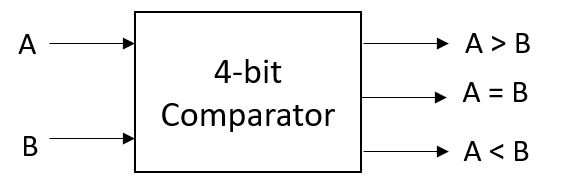Tutorials

A comparator has two inputs and three output bits that say whether the first input is greater, less, or equal to the second input.

## 4 Bit Comparator

### Block Diagram### 4 Bit Comparator Verilog Code

``````module comparator(
input [3:0] A, B,
output reg A_grt_B, A_less_B, A_eq_B);

always@(*) begin
A_grt_B = 0; A_less_B = 0; A_eq_B = 0;
if(A>B) A_grt_B = 1'b1;
else if(A<B) A_less_B = 1'b1;
else A_eq_B = 1'b1;
end
endmodule``````

### Testbench Code

``````module tb;
reg [3:0] A, B;
wire A_grt_B, A_less_B, A_eq_B;

comparator comp(A, B, A_grt_B, A_less_B, A_eq_B);

initial begin
\$monitor("A = %0h, B = %0h -> A_grt_B = %0b, A_less_B = %0b, A_eq_B = %0b", A, B, A_grt_B, A_less_B, A_eq_B);
repeat(5) begin
A=\$random; B=\$random; #1;
end
end
endmodule``````

Output:

``````A = 4, B = 1 -> A_grt_B = 1, A_less_B = 0, A_eq_B = 0
A = 9, B = 3 -> A_grt_B = 1, A_less_B = 0, A_eq_B = 0
A = d, B = d -> A_grt_B = 0, A_less_B = 0, A_eq_B = 1
A = 5, B = 2 -> A_grt_B = 1, A_less_B = 0, A_eq_B = 0
A = 1, B = d -> A_grt_B = 0, A_less_B = 1, A_eq_B = 0``````

Verilog Codes